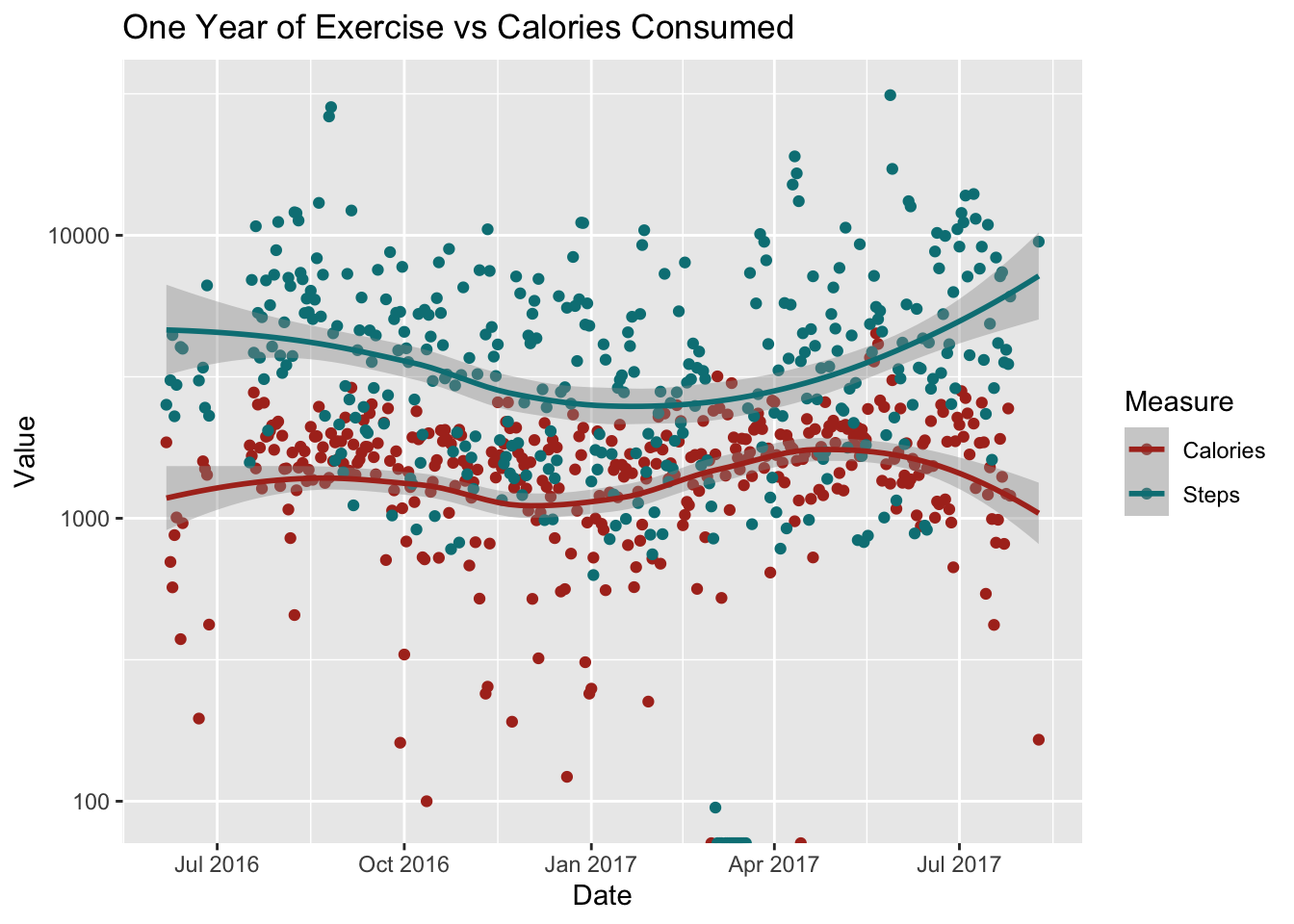# Richard Sprague

My personal website

# My activity data

Created: 2015-07-23 ; Updated: 2015-07-23

I’ve carefully tracked all my food and exercise for a year. Now I want to display, and then analyze it to see if I can find patterns.

Let’s look at the overall picture:

activity <- tbl_df(with(rikActivity,data.frame(Date=Start,Steps=Steps (count))))
## Warning: tbl_df() is deprecated as of dplyr 1.0.0.
## Please use tibble::as_tibble() instead.
## This warning is displayed once every 8 hours.
## Call lifecycle::last_warnings() to see where this warning was generated.
activity$Date <- as.Date(activity$Date)
rik.calories <- rikfood %>% select(Date,Calories) %>% group_by(Date) %>% summarize(Calories = sum(Calories))
## summarise() ungrouping output (override with .groups argument)

df <- na.omit(rik.calories[rik.calories$Date %in% as.Date(activity$Date),])

#activity %>% dplyr::filter(Date %in% df$Date) %>% group_by(Date) %>% summarize(Steps = sum(Steps)) df2 <- left_join(activity %>% dplyr::filter(Date %in% df$Date) %>% group_by(Date) %>% summarize(Steps = sum(Steps)),
df)
## summarise() ungrouping output (override with .groups argument)
## Joining, by = "Date"

df <- df2
#df$Steps <- na.omit(activity[as.Date(activity$Date) %in% rik.calories$Date ,])[["Steps"]] rik.motion <- data.frame(Date = df$Date, Calories = df$Calories, Steps = df$Steps)

ggplot(data=rik.motion %>% gather(Measure,Value,-Date), aes(x=Date,y=Value,color=Measure)) +
geom_point() + geom_smooth(method = "loess") +
theme(legend.position = "right") + scale_color_hue(l = 40) + scale_y_log10() +
#  annotate("pointrange",x = kefir.day, y = 100, ymin = 100, ymax = 110, color = "blue", size = 0.1) +
#  annotate("text",y = 150, x =as.Date("2017-04-10"),  label = "Dates I drank kefir", color = "blue") +
ggtitle("One Year of Exercise vs Calories Consumed")
## Warning: Transformation introduced infinite values in continuous y-axis
## Warning: Transformation introduced infinite values in continuous y-axis
## geom_smooth() using formula 'y ~ x'
## Warning: Removed 16 rows containing non-finite values (stat_smooth).